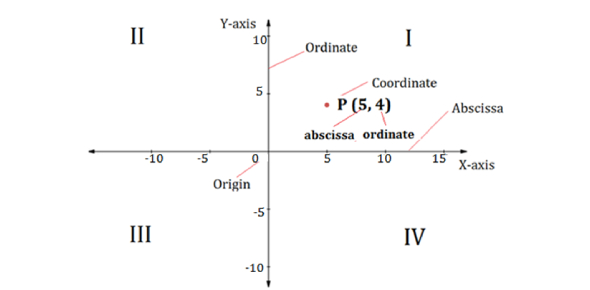# Coordinate Geometry MCQ Test: Trivia!

15 Questions | Attempts: 4007
ShareSettings.

• 1.
The distance of the point P (2,3) from the x-axis is:
• A.

2

• B.

5

• C.

3

• D.

1

• 2.
The points (-4,0), (4,0), (0,3) are the vertices of a:
• A.

Right Triangle

• B.

Isosceles Triangle

• C.

Equilateral Triangle

• D.

Scalene Triangle

• 3.
The distance between the points A (0,6) and B (0,-2) is:
• A.

6

• B.

8

• C.

4

• D.

2

• 4.
The area of a triangle with vertices A (3,0), B (7,0) and C (8,4) is:
• A.

14

• B.

28

• C.

8

• D.

6

• 5.
Point A (-5,6) is at a distance of:
• A.

units from origin

• B.

units from origin

• C.

61 units from origin

• D.

11 units from origin

• 6.
AOBC is a rectangle whose three vertices are A (0,8), O(0,0), and B (6,0). The length of its diagonal is:
• A.

10

• B.

9

• C.

8

• D.

4

• 7.
If the points (1,x), (5,2) and (9,5) are collinear then value of x is:
• A.

5/2

• B.

-5/2

• C.

-1

• D.

1

• 8.
The perimeter of a triangle with vertices (0,4), (0,0) and (3,0) is:
• A.

4

• B.

5

• C.

7

• D.

12

• 9.
Point on y-axis has coordinates:
• A.

(-a,b)

• B.

(a,0)

• C.

(0,b)

• D.

(-a,-b)

• 10.
The endpoints of the diameter of a circle are (2,4) and (-3,-1). The radius of the circle is:
• A.
• B.
• C.
• D.
• 11.
The point which lies on the perpendicular bisector of the line segment joining the points A (-2, -5) and B (2, 5) is:
• A.

(-2, 0)

• B.

(0, 2)

• C.

(2, 0)

• D.

(0, 0)

• 12.
The ratio in which x-axis divides the line segment joining the points (5,4) and (2,-3) is:
• A.

5:2

• B.

3:4

• C.

2:5

• D.

4:3

• 13.
The vertex D of a parallelogram ABCD whose three vertices are A (-2, 3), B (6, 7) and C (8, 3) is:
• A.

(0, 1)

• B.

(0, -1)

• C.

(-1, 0)

• D.

(1 ,0)

• 14.
The point which divides the line segment joining the points (7,-6) and (3,4) in ratio1 : 2 internally lies in the:
• A.

• B.

• C.

• D.

• 15.
If the point P (2, 1) lies on the line segment joining points A (4, 2) and B (8, 4), then:
• A.

AP = 1/3 AB

• B.

AP = PB

• C.

PB = 1/3 AB

• D.

AP = 1/2 AB

## Related TopicsBack to top
×

Wait!
Here's an interesting quiz for you.